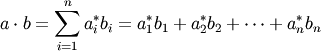# numpy.vdot¶

numpy.vdot(a, b)

Return the dot product of two vectors.

The vdot(a, b) function handles complex numbers differently than dot(a, b). If the first argument is complex the complex conjugate of the first argument is used for the calculation of the dot product.

For 2-D arrays it is equivalent to matrix multiplication, and for 1-D arrays to inner product of vectors (with complex conjugation of a). For N dimensions it is a sum product over the last axis of a and the second-to-last of b:

dot(a, b)[i,j,k,m] = sum(a[i,j,:] * b[k,:,m])

Parameters: a : array_like If a is complex the complex conjugate is taken before calculation of the dot product. b : array_like Second argument to the dot product. output : ndarray Returns dot product of a and b. Can be an int, float, or complex depending on the types of a and b.

dot
Return the dot product without using the complex conjugate of the first argument.

Notes

The dot product is the summation of element wise multiplication.Examples

>>> a = np.array([1+2j,3+4j])
>>> b = np.array([5+6j,7+8j])
>>> np.vdot(a, b)
(70-8j)
>>> np.vdot(b, a)
(70+8j)
>>> a = np.array([[1, 4], [5, 6]])
>>> b = np.array([[4, 1], [2, 2]])
>>> np.vdot(a, b)
30
>>> np.vdot(b, a)
30


numpy.dot

numpy.inner